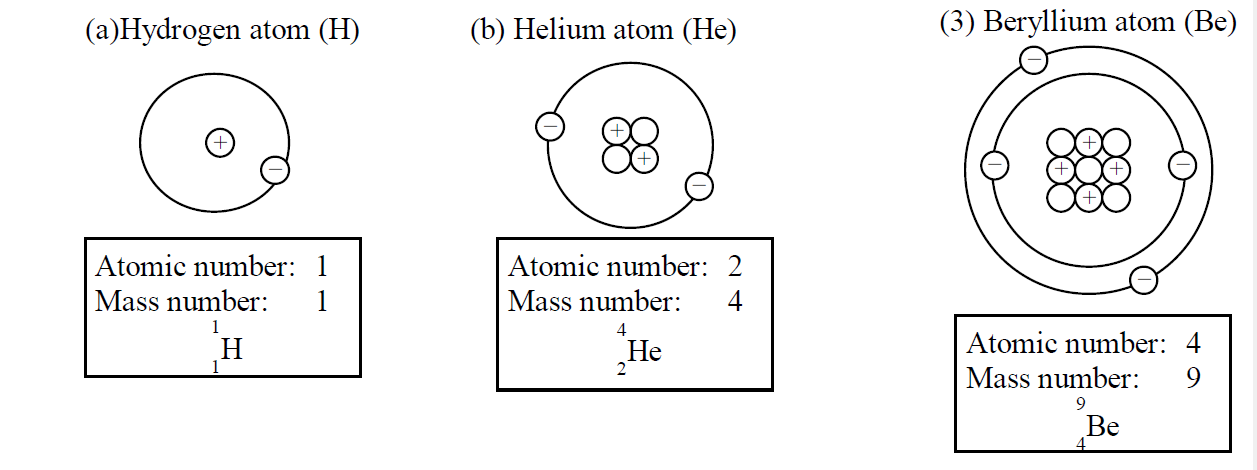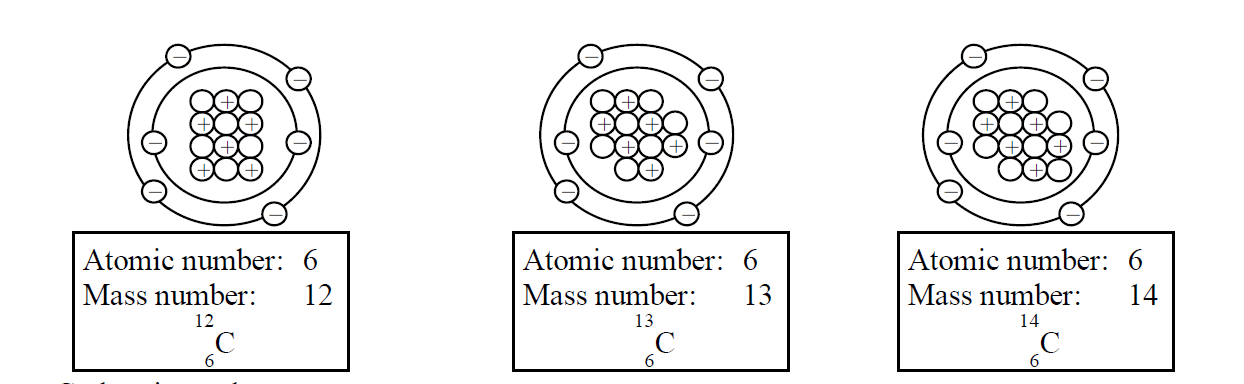# Atomic Physics

#### Nucleus

##### Composition of atom

- An atom consists of proton , neutron and electron .

- A Proton is positivery charged

- An electron is negatively charged.

- A neutron is neutral.

- Protons and neutrons are bound together in the nucleus .

- Protons and neutrons is called the nucleon.

- Electrons move round the nucleus in different orbits called shells .

- Electrons and protons carry equal numbers in an atom.

- If atom is neutral, the number of electrons is the same as the number of protons.

#### Atomic number and Mass number

- The number of protons in an atom is called the atomic number or proton number (Z).

- The total number of nucleons in a nucleus is called the mass number or nucleon number (A).

- If the number of neutrons in the nucleus is N,

Formula for Mass number

$A=Z + N$

A: Mass number

Z: Atomic number

N: Number of neutrons

An element of chemical symbol X with a mass number A and an atomic number Z is expressed,

#### Example

Express the following elements by the symbol of ${}^{A}{}_{Z}X$

Solution#### Nuclide and Isotopes

Each different form of nucleus is called a Nuclide .

Atoms which have the same atomic number but different mass numbers are called Isotopes of an element.- Carbon is an element.